# Electromagnetism and a solid metallic sphere

## Homework Statement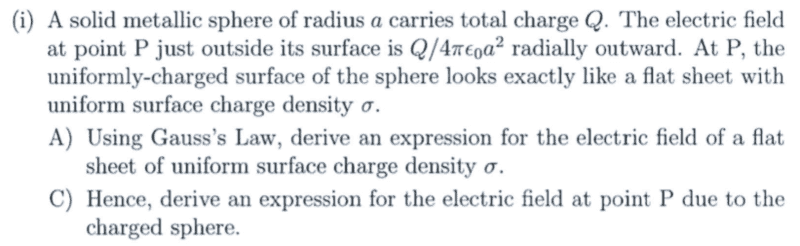## Homework Equations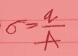## The Attempt at a Solution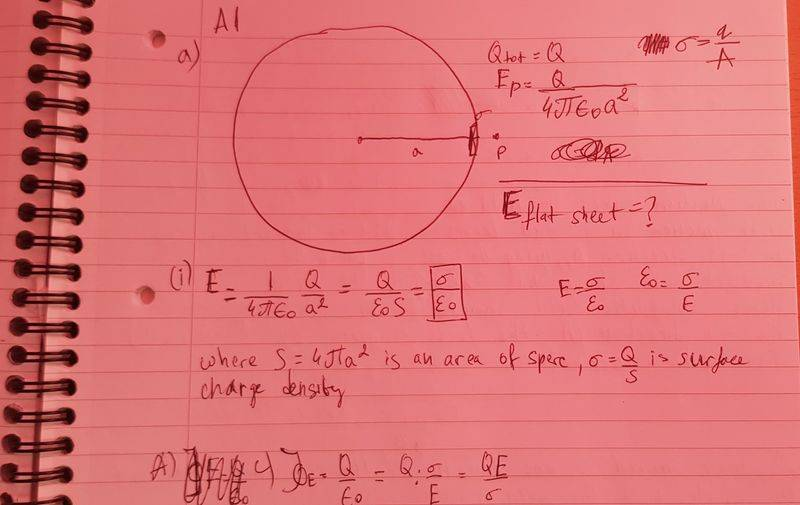The textbook says that the electric field on a surface of a conductor is: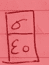. So, I guess since the sphere is metallic I can assume that what I have written there is true?

#### Attachments

Homework Helper
Gold Member
The answer for the electric field ## E ## of a flat sheet with surface charge density ## \sigma ## needs some qualification here. A surface charge density of ## \sigma ## gives the result by Gauss' law that ## E=\frac{\sigma}{\epsilon_o} ## if there is a conductor on one side, but otherwise the result is ## E=\frac{\sigma}{2 \epsilon_o} ## if there is free space on both sides. It is the first case that applies here. ## \\ ## Gauss' law says that the electric flux which is ## \int E \cdot dA=\frac{Q_{enclosed}}{\epsilon_o} ##. It is difficult to explain the idea of flux without a chalkboard, but perhaps what I have given you will be helpful. ## \\ ## If you understand Gauss' law, deriving the result that ## E=\frac{\sigma}{\epsilon_o} ## at the surface of a conductor is very straightforward.

•Jozefina Gramatikova
The answer for the electric field ## E ## of a flat sheet with surface charge density ## \sigma ## needs some qualification here. A surface charge density of ## \sigma ## gives the result by Gauss' law that ## E=\frac{\sigma}{\epsilon_o} ## if there is a conductor on one side, but otherwise the result is ## E=\frac{\sigma}{2 \epsilon_o} ## if there is free space on both sides. It is the first case that applies here. ## \\ ## Gauss' law says that the electric flux which is ## \int E \cdot dA=\frac{Q_{enclosed}}{\epsilon_o} ##. It is difficult to explain the idea of flux without a chalkboard, but perhaps what I have given you will be helpful. ## \\ ## If you understand Gauss' law, deriving the result that ## E=\frac{\sigma}{\epsilon_o} ## at the surface of a conductor is very straightforward.
what do you mean by "if there is free space on both sides"?

Homework Helper
Gold Member
what do you mean by "if there is free space on both sides"?
A layer of surface charge where you have air on both sides when you draw what is called a Gaussian pillbox around a section of area ## A ## of surface charge density ## \sigma ##. When you calculate the flux on the "inside", the conductor necessarily has ## E=0 ## inside the conductor, so there is no contribution to the flux from the inside surface. Alternatively, if there is air on both sides, both sides get the same outward pointing ## E ## and the result is ## E=\frac{\sigma}{2 \epsilon_o} ##. ## \\ ## (In full detail, for the conductor case: ## \int E \cdot dA=EA=\frac{\sigma A}{\epsilon_o} ##, so that ## E=\frac{\sigma}{\epsilon_o} ##. ## \\ ## And for the case with air on both sides: ## \int E \cdot dA=2 E A=\frac{\sigma A}{\epsilon_o } ##, so that ## E=\frac{\sigma}{2 \epsilon_o} ## ).

Last edited:
•Jozefina Gramatikova
A layer of surface charge where you have air on both sides when you draw what is called a Gaussian pillbox around a section of area ## A ## of surface charge density ## \sigma ##. When you calculate the flux on the "inside", the conductor necessarily has ## E=0 ## inside the conductor, so there is no contribution to the flux from the inside surface. Alternatively, if there is air on both sides, both sides get the same outward pointing ## E ## and the result is ## E=\frac{\sigma}{2 \epsilon_o} ##. ## \\ ## (In full detail, for the conductor case: ## \int E \cdot dA=EA=\frac{\sigma A}{\epsilon_o} ##, so that ## E=\frac{\sigma}{\epsilon_o} ##. ## \\ ## And for the case with air on both sides: ## \int E \cdot dA=2 E A=\frac{\sigma A}{\epsilon_o } ##, so that ## E=\frac{\sigma}{2 \epsilon_o} ## ).
Oh, damn. I finally understood it! Thank you so much! It actually makes sense!

•A layer of surface charge where you have air on both sides when you draw what is called a Gaussian pillbox around a section of area ## A ## of surface charge density ## \sigma ##. When you calculate the flux on the "inside", the conductor necessarily has ## E=0 ## inside the conductor, so there is no contribution to the flux from the inside surface. Alternatively, if there is air on both sides, both sides get the same outward pointing ## E ## and the result is ## E=\frac{\sigma}{2 \epsilon_o} ##. ## \\ ## (In full detail, for the conductor case: ## \int E \cdot dA=EA=\frac{\sigma A}{\epsilon_o} ##, so that ## E=\frac{\sigma}{\epsilon_o} ##. ## \\ ## And for the case with air on both sides: ## \int E \cdot dA=2 E A=\frac{\sigma A}{\epsilon_o } ##, so that ## E=\frac{\sigma}{2 \epsilon_o} ## ).
So, the solution to part A) is: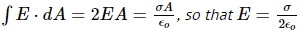and part C) is: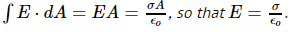?

#### Attachments

Homework Helper
Gold Member
So, the solution to part A) is: View attachment 229715
and part C) is: View attachment 229716 ?
Part A needs to be described in more detail, and they need to specify "with a conductor on one side". Without that additional detail being included, part C does not follow. I do think this my have been an oversight on the part of the person who made up the question.

Part A needs to be described in more detail, and they need to specify "with a conductor on one side". Without that additional detail being included, part C does not follow. I do think this my have been an oversight on the part of the person who made up the question.
It says it is a metallic sphere so isn't it a conductor?

Homework Helper
Gold Member
It says it is a metallic sphere so isn't it a conductor?
Yes, that means it's a conductor, but they did not tell you in part A to derive the Gauss' law for the case of conductor material on one side of the Gaussian pillbox. It is a very good result that they are having you derive, but they need to present the question with sufficient detail that you get the correct result. There are two different cases that can occur. The case with air on both sides is not relevant here, but if you just read part A, it would appear that's what they are asking for.

•Jozefina Gramatikova
Yes, that means it's a conductor, but they did not tell you in part A to derive the Gauss' law for the case of conductor material on one side of the Gaussian pillbox. It is a very good result that they are having you derive, but they need to present the question with sufficient detail that you get the correct result. There are two different cases that can occur. The case with air on both sides is not relevant here, but if you just read part A, it would appear that's what they are asking for.
I see. Thank you!

•